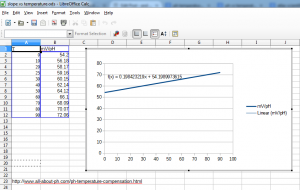Introduction

Atlas Scientific makes a nice pH circuit that is very simple to setup and communicate with via serial.

Part of my Aquarium Monitoring project, I decided to employ 4 pH Circuits (v5.0) to retrieve the pH in all my 4 aquariums. I encountered two problems:
a) The Multi Circuit Carrier Board (that allows connecting 4 pH Circuits on a ready made board with BNC connectors) doesn’t give the possibility to hookup a PWR ISO individually for each channel.
b) There’s no possibility to access the mV values recorded by the device, you only get a string representing the pH value.

I will describe the impact of problem a) in a future post.
Impact of problem b) is that without access to the mV values:
1) you can’t determine the slope of the electrode (so that you can tell how healthy it is). An electrode is considered good if the ph 7 offset is within the +/-25mV and the slope is between 90% and 105%.
2) no possibility to do software calibration given the raw mV values (so that you can use pH buffers of 7, 4 and 9 for example)

My controller is a pcDuino3 since I believe Arduino shields are much more expensive than needed so with a pcDuino3 I got a smart node which aggregates all measured values (for now pH and temperatures using DS18B20 sensors) to a Cacti server for MRTG charts of the values.

I had difficulties finding a pH 10 buffer solution to calibrate the pH Circuit at the 7 4 and 10 values. You can’t tell it to calibrate at 9 for example, but if you could get access to the raw mV values of the electrode, then you could calculate the offset and the slope (and do a proper regression line) using other pH buffers beside 4 or 10.

I decided to try to work out the mV from the output of pH Circuit.

Equations:

Since the pH Circuit offers an output even after being reset (the X command) it meant it has a function (either hardware or software) that will take the mV output of the electrode and convert it to a pH value, considering the temperature to be 25C.

a) in the reset state, the pH circuit assumes the electrode is ideal, and that a 0 mV readout from the electrode will be shown as ph “7.00” (and for example, a ~170mV output of the electrode will be shown as “4.00”).
b) in the reset state, the pH Circuit assumes the slope to be 59.15 mV/pH (pH Circuit documentation says that it will consider the temperature of 25C once reset).

Thus:

However, there was no way I could test that this function holds.

I got curious if how the pH Circuit adjusts the output values when you send various temperature commands. Since I’m currently testing with two aquariums, one had a stable pH at 7.86 and the second one had the pH at around 6.92 (both pH Circuits were in the reset state / no calibration).

I mapped out the output of pH Circuits after sending it various temperatures commands:

So for the Tank 1, the output decreased with 0.03 pH units per each 10C, while for Tank 2 it almost stayed the same. While I sent the commands (let’s say everything took less than few minutes) the tanks were during lights off thus I assumed there were no temperature / pH variations that could change the output of the electrodes themselves.

After reading more about the pH electrode mV corrections related to temperature, it was normal to have a 0.03 increments when the pH is at 8, and almost no influence when the pH is 7). And this is what the pH Circuit was reporting! So it behaves correctly.

I found a table of the slope dependency to temperature here: http://www.all-about-ph.com/ph-temperature-compensation.html and put it in Libre Office to see the chart:It was linear. I asked Libre Office to insert a regression line for the slope to temperature table and the rounded up function was

Where T is in Celsius and f(T) represents the slope value depending on the temperature.

Putting the slope into the initial mV equation results in:

where:

T is the temperature in Celsius and pH is the pH circuit output.

So now I had an electrode which was at 25C reporting “6.92” value, what should pH Circuit display if we assume the temperature is actually 0C ?

At 25C, and output of 6.92, then with equation 1:

Since the temperature of the tank / electrode isn’t actually changed (so still the same mV value is read from the electrode) by pH Circuit, we could work out what value is should display based on the slope:

This is exactly the value reported by pH Circuit when I send it the “00.00” command (asking it to assume a temperature of 0C) 🙂

So now I can estimate the mV of the electrode given the temperature of the tank is known (but I use a DS18B20 isolated sensor for each tank) and the output of a uncalibrated pH Circuit.

References: